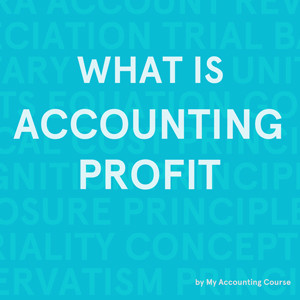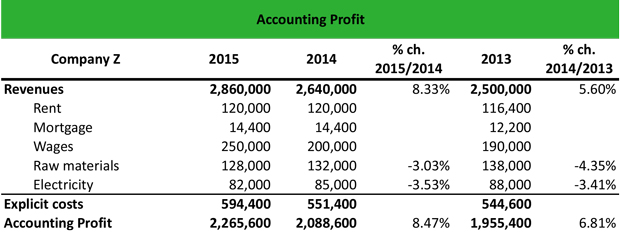# What is Accounting Profit?

Definition: Accounting profit, also called bookkeeping profit, is the net income that remains after subtracting the explicit costs from a firm’s total revenues in accordance with GAAP. These costs include labor costs, raw material costs, distribution costs, and other production expenses.

## What Does Accounting Profit Mean?

What is the definition of accounting profit? This is the net income reported on the all GAAP basis financial statements. Accountants subtract a firm’s explicit costs from the total revenues to calculate the accounting profit. Explicit costs are costs that can be clearly identified and measured. For example, labor costs are explicit costs because they represent a specific amount paid for wages during a given period.All of the costs included in the calculation are amounts actually paid except depreciation expense. This represents the year’s ratable portion of the past outlay of cash required to purchase production equipment. Thus, this also is included.

Accountants do not consider implicit costs in this calculation because they haven’t been incurred and are merely theoretical. Implicit costs are used for the calculation of a firm’s economic profit.

Let’s look at an example.

## Example

Mario, the chief accountant of a manufacturing company that sells air conditioners, asks his assistant to calculate the firm’s accounting profit over the past three years. Mario wants to confirm that the profit of the company is increasing, indicating that the company is effectively managing its costs.

The assistant creates an excel file with all the information he has for the firm’s operations in 2013, 2014, and 2015, i.e. total revenues and explicit costs (rent, mortgage, wages, raw materials and electricity expenses).The assistant finds that the profit for the company in the fiscal year 2015 is \$2.27 million, for 2014 is \$2.09 million and for 2013 is \$1.96 million. The increase of 8.47% YoY from 2014 to 2015 and of 6.81% YoY from 2013 to 2014 indicates that the firm implements effective strategies to lower its explicit costs and increase its revenues. Specifically, the revenue growth from 2013 to 2014 is 5.60% YoY and from 2014 to 2015 is 8.33% YoY.

Furthermore, the company has lowered its raw materials costs by 4.35% YoY from 2013 to 2014 and by 3.03% YoY from 2014 to 2015. Likewise, the electricity costs are reduced by 3.53% YoY from 2013 to 2014 and by 3.53% YoY from 2014 to 2015.

## Summary Definition

Define Accounting Profit: Accounting profit is the GAAP concept of where total revenue exceeds explicit costs.